# Dusty Hydromagnetic Oldryod Fluid Flow in a Horizontal Channel with Volume Fraction and Energy Dissipation

Dusty Hydromagnetic Oldryod Fluid Flow in a Horizontal Channel with Volume Fraction and Energy Dissipation

Debasish Dey

Department of Mathematics, Dibrugarh University, Dibrugarh-786 004, India

Corresponding Author Email:
debasish41092@gmail.com
Page:
415-422
|
DOI:
https://doi.org/10.18280/ijht.340310
|
Accepted:
|
Published:
30 September 2016
| Citation

OPEN ACCESS

Abstract:

An unsteady magneto-hydrodynamics flow of dusty Oldroyd fluid through a horizontal channel has been investigated under the influence of dissipation of energy and volume fraction. This energy dissipation generates the mechanism of heat transfer in the governing fluid motion and the dust particles absorb the heat through conduction. To study the dusty-visco-elastic fluid flow, Saffman model and Oldroyd model have been used. The visco-elastic responses are exhibited through the two rheological parameters $\lambda_1$ & $\lambda_2$ (relaxation time and retardation time). To restrain the weak turbulent motion, a magnetic field of strength B0 is applied along the transverse direction to the plate. The lower plate of the horizontal channel is kept fixed but the upper one is oscillating with the velocity $U_0(1+εe^{iω't'})$. The governing equations of motion are solved analytically and the results are discussed graphically/ numerically for various values of flow parameters involved in the solution.

Keywords:

Oldroyd fluid, Saffman model, Nusselt number, Volume fraction, Relaxation and retardation.

1. Introduction

Oldroyd [1, 2] has formulated the constitutive model to study the flow behaviour of visco-elastic fluids having two rheological parameters as relaxation time and retardation times characterizing the response of visco-elastic material. Sellers and Walker  have investigated the problem of liquid metal in an electrically insulated rectangular duct with a non-uniform magnetic field. Visco-elastic fluid flow past porous surface due to fluctuation in main flow has been analyzed by Mukhopadhyay and Chaudhury . Rajagopal and Bhatnagar , Ray et al.  gave the exact solution of Oldroyd fluid flows. Exact solutions of (i) stokes problem, (ii) modified stokes problem, (iii) the time-periodic Poiseuille fow due to an oscillating pressure gradient (iv) the non-periodic fows between two boundaries, and (v) symmetric flow with an arbitrary initial velocity using Oldroyd model have been obtained by Hayat et al. . Motion of electrically conducting, Oldroyd-B fluid between two non-conducting parallel plates in a rotating system under uniform transverse magnetic field has been studied by Hayat et al. . Hall effects on unsteady fluid flows governed by Oldroyd-B model have been analyzed by Asghar et al.  and Hayat et al. . Effect of Oldroyd fluid on unsteady free convective flow through porous medium along a moving porous hot vertical plate in presence of heat and mass transfer has been studied by Prasad et al. . Unsteady hydro-magnetic flow of an Oldroyd fluid through a porous channel with oscillating walls using Laplace transform method has been examined by Ghosh . Choudhury and Das , Choudhury Purkayastha , Choudhury and Das  have studied hydromagnetic visco-elastic fluid flow with various physical properties.

Application of visco-elastic fluids may be seen in various chemical and nucleaus industries, material processing, geophysics and in medical science. Flow problem of dusty visco-elastic fluid with heat transfer in presence of magnetic field may be applied in the extrusion of polymer sheet from a die. Polymers are actually mixture of various organic solutions, so they may be modeled as visco-elastic fluid model (for fluid phase) and Saffman model (for dust phase). Another important application of dusty visco-elastic fluid flow model is  in blood flow. In blood flow, some parts of energy transferred by heart are stored due to elasticity, some parts are transformed into heat by viscosity and remaining energy is used in motion of blood . Viscosity in combination with elasticity plays an important role in blood flow. In this paper, an unsteady electrically conducting dusty visco-elastic flow characterized by Oldroyd fluid model through horizontal channel has been considered in presence of volume fraction and energy dissipation.

2. Mathematical Formulation

An unsteady flow of dusty electrically conducting Oldroyd fluid in a horizontal channel has been considered. The channel is bounded by two parallel plates, the lower one is kept fixed and the upper one is oscillating with velocity $u^{\prime}=U_{0}\left(1+\varepsilon e^{i \omega \prime t^{\prime}}\right)$ , $U_{0}$  is a constant and they are kept at different temperatures T1 and $T_{2}+\varepsilon\left(T_{2}-T_{1}\right) e^{i \omega^{\prime} t^{\prime}}$ (T2 > T1). The upper plate is oscillating about the mean temperature $T_{2}$ . Dust particles are assumed to be electrically non-conducting, spherical in shape and uniformly distributed throughout the fluid. A magnetic field of uniform strength B0 is applied along the transverse direction to the plate.

## figure_1.png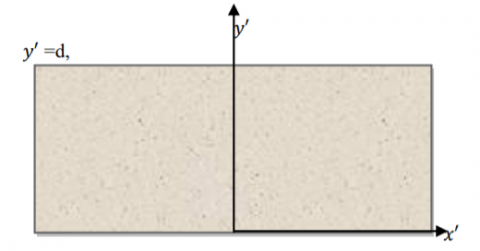Figure 1. Physical description of the Problem

Equation of Continuity:

$\mathrm{v}_{\mathrm{i}, \mathrm{i}}=0$(1)

Equation of Continuity for dust particles:

$\mathrm{v}_{\mathrm{pi}, \mathrm{i}}=0$(2)

Momentum Equation:

$\rho\left[\frac{\partial \mathrm{v}_{\mathrm{i}}}{\partial \mathrm{t}}+2 \mathrm{v}_{\mathrm{k}} \frac{\partial \mathrm{v}_{\mathrm{i}}}{\partial \mathrm{x}_{\mathrm{k}}}\right]=-\mathrm{p}_{\mathrm{i}}+\tau_{\mathrm{i} \mathrm{j}, \mathrm{j}}+\varepsilon_{\mathrm{ijk}} \mathrm{J}_{\mathrm{j}} \mathrm{B}_{\mathrm{k}}+\frac{\mathrm{KN}}{1-\phi}\left(\mathrm{v}_{\mathrm{pi}}-\mathrm{v}_{\mathrm{i}}\right)$(3)

Momentum equation for dust particles:

$\mathrm{m}_{\mathrm{p}}\left[\frac{\partial \mathrm{v}_{\mathrm{pi}}}{\partial \mathrm{t}}+\mathrm{v}_{\mathrm{pk}} \frac{\partial \mathrm{v}_{\mathrm{pi}}}{\partial \mathrm{x}_{\mathrm{k}}}\right]=\frac{\phi}{\rho}\left(-\mathrm{p}_{\mathrm{i}}+\tau_{\mathrm{ij}, \mathrm{j}}+\varepsilon_{\mathrm{ijk}} \mathrm{J}_{\mathrm{j}} \mathrm{B}_{\mathrm{k}}\right)-\mathrm{K}\left(\mathrm{v}_{\mathrm{pi}}-\mathrm{v}_{\mathrm{i}}\right)$(4)

Constitutive Equation:

$\left(1+\lambda_{1} \frac{d}{d t}\right) \tau_{i k}=2 \eta_{0}\left(1+\lambda_{2} \frac{d}{d t}\right) \epsilon^{i k}$(5)

where, $\lambda_{1}$ and $\lambda_{2}$  denote relaxation and retardation times respectively. $\left(\lambda_{1}=0, \lambda_{2}=0\right)$ characterizes Newtonian fluid, $\left(\lambda_{1}=0, \lambda_{2} \neq 0\right)$  characterizes Second-grade fluid and  $\left(\lambda_{1} \neq 0, \lambda_{2}=0\right)$ represents the Maxwell fluid model.

Energy Equation:

$\rho C_{p} \frac{\partial T^{\prime}}{\partial t^{\prime}}=k T_{, i i}^{\prime}+\tau_{i j} v_{i, j}+\frac{\rho_{p} C_{s}}{\gamma_{T}}\left(T_{p}^{\prime}-T^{\prime}\right)$(6)

Following Attia et al. , energy Equation for dust particles:

$\frac{\partial T^{\prime} p}{\partial t^{\prime}}=\frac{1}{\gamma_{T}}\left(T^{\prime}-T_{p}^{\prime}\right)$(7)

2.1 Velocity distribution

For an incompressible unsteady flow through a horizontal channel, the stress components derived from (5) are obtained as follows:

$\tau_{x x}^{\prime}+\lambda_{1}\left(\frac{\partial}{\partial t^{\prime}} \tau_{x x}^{\prime}-\frac{\partial u^{\prime}}{\partial y^{\prime}} 2 \tau_{y x}^{\prime}\right)=\frac{-\eta_{0} \lambda_{2}}{2}\left(\frac{\partial u^{\prime}}{\partial y^{\prime}}\right)^{2}$(8)

$\tau_{y x}^{\prime}+\lambda_{1}\left(\frac{\partial}{\partial t^{\prime}} \tau_{x y}^{\prime}-\frac{\partial u^{\prime}}{\partial y^{\prime}} \tau_{y y}\right)=\eta_{0}\left[1+\lambda_{2}\left(\frac{\partial}{\partial t^{\prime}}\right)\right] \frac{\partial u^{\prime}}{\partial y^{\prime}}$(9)

$\tau_{y y}+\lambda_{1}\left(\frac{\partial}{\partial t} \tau_{y y}\right)=0$(10)

Solving (10), we get $\tau_{y y}=0$  [8, 10] and the governing equations of motion are:

$\frac{\partial u^{\prime}}{\partial t^{\prime}}=-\frac{1}{\rho} \frac{\partial p^{\prime}}{\partial x^{\prime}}+\frac{1}{\rho} \frac{\partial \tau_{x y}^{\prime}}{\partial y^{\prime}}-\frac{\sigma B_{0}^{2} u^{\prime}}{\rho}+\frac{K N}{1-\phi}\left(v^{\prime}-u^{\prime}\right)$(11)

$\frac{\partial v^{\prime}}{\partial t^{\prime}}=-\frac{\phi}{\rho m_{p}}\left[\frac{\partial p^{\prime}}{\partial x^{\prime}}-\frac{\partial \tau_{x y}^{\prime}}{\partial y^{\prime}}+\sigma B_{0}^{2} u^{\prime}\right]-K N\left(v^{\prime}-u^{\prime}\right)$(12)

The boundary conditions of the problem are

$y=0$: $u'=0$, $v'=0$;

$y=d$: $u'=U(t)=U_0(1+εe^{iω't'})=v'$  (13)

Using the constitutive equations, the equations (11) and (12) are written as follows:

$\left(1+\lambda_{1} \frac{\partial}{\partial t^{\prime}}\right) \frac{\partial u^{\prime}}{\partial t^{\prime}}=-\frac{1}{\rho}\left(1+\lambda_{1} \frac{\partial}{\partial t^{\prime}}\right) \frac{\partial p^{\prime}}{\partial x^{\prime}}+\frac{1}{\rho} \eta_{0}\left[\frac{\partial u^{\prime}}{\partial y^{\prime}}+\lambda_{2}\left(\frac{\partial^{2} u^{\prime}}{\partial y^{\prime} \partial t}\right)\right]-\frac{\sigma B_{0}^{2}}{\rho}\left(1+\lambda_{1} \frac{\partial}{\partial t^{\prime}}\right) u^{\prime}+\frac{K N}{1-\phi}\left(1+\lambda_{1} \frac{\partial}{\partial t^{\prime}}\right)\left(v^{\prime}-u^{\prime}\right)$(14)

$\left(1+\lambda_{1} \frac{\partial}{\partial t^{\prime}}\right) \frac{\partial v^{\prime}}{\partial t^{\prime}}=\frac{\phi}{m_{p}}\left[-\frac{1}{\rho}\left(1+\lambda_{1} \frac{\partial}{\partial t^{\prime}}\right) \frac{\partial p^{\prime}}{\partial x^{\prime}}+\frac{1}{\rho} \eta_{0}\left\{\frac{\partial u^{\prime}}{\partial y^{\prime}}+\lambda_{2}\left(\frac{\partial^{2} u^{\prime}}{\partial y^{\prime} \partial t}\right)\right\}-\frac{\sigma B_{0}^{2}}{\rho}\left(1+\lambda_{1} \frac{\partial}{\partial t^{\prime}}\right) u^{\prime}\right]+\frac{K N}{m_{p}}\left(1+\lambda_{1} \frac{\partial}{\partial t^{\prime}}\right)\left(u^{\prime}-v^{\prime}\right)$(15)

The pressure gradient terms are eliminated by using boundary conditions (13) and it is observed that

$-\frac{1}{ρ}(1+λ_1\frac{∂}{∂t'})\frac{∂p'}{∂x'}=(A_1+iA_2)e^{iω't'}$   (16)

where, $A_{1}$ and $A_{2}$ are arbitrary constants.

Using (16) in (14) and (15), we get

$\left(1+\lambda_{1} \frac{\partial}{\partial t^{\prime}}\right) \frac{\partial u^{\prime}}{\partial t^{\prime}}=\left(A_{1}+i A_{2}\right) e^{i \omega^{\prime} t^{\prime}}+\frac{1}{\rho} \eta_{0}\left[\frac{\partial u^{\prime}}{\partial y^{\prime}}+\lambda_{2}\left(\frac{\partial^{2} u^{\prime}}{\partial y^{\prime} \partial t}\right)\right]-\frac{\sigma B_{0}^{2}}{\rho}\left(1+\lambda_{1} \frac{\partial}{\partial t^{\prime}}\right) u^{\prime}+\frac{K N}{1-\phi}\left(1+\lambda_{1} \frac{\partial}{\partial t^{\prime}}\right)\left(v^{\prime}-u^{\prime}\right)$(17)

$(1+λ_1\frac{∂}{∂t'})$$\frac{∂v'}{∂t'}=$$\frac{ϕ}{m_p}$$[(A_1+iA_2)e^{iω't'}+\frac{1}{ρ}η_0{\frac{∂u'}{∂y'}+λ_2(\frac{∂^2u'}{∂y'∂t})}$$-\frac{σB_0^2}{ρ}$$(1+λ_1\frac{∂}{∂t'})u']$$+\frac{KN}{m_p}(1+λ_1\frac{∂}{∂t'})(u'-v')$   (18)

Let us introduce the following non-dimensional quantities

$u=\frac{u'}{U_0}$, $v=\frac{v'}{U_0}$, $y=\frac{y'}{d}$, $\frac{t}{t'}=\frac{U_0}{d}$, $\frac{ω}{ω'}=\frac{d}{U_0}$, $R=\frac{U_0d}{ν}$, $α_1=\frac{λ_1U_0}{d}$

$α_2=\frac{λ_2U_0}{d}, M=\frac{σB_0^2d}{ρU_0}, ϵ_1=\frac{1}{1-ϕ}, f=\frac{KNd^2}{η_0}, G=\frac{m_pη_0}{KNd^2}$ (19)

Using the above mentioned non-dimensional quantities in (17) and (18), we get the following dimensionless equations of motion,

$\frac{∂u}{∂t}+α_1\frac{∂^2u}{∂t^2}=(A_1+iA_2)e^{iωt}+\frac{1}{R}(\frac{∂^2u}{∂y^2}+α_2\frac{∂^3u}{∂y^2∂t})-\frac{1}{R}(1+α_1\frac{∂}{∂t})[Mu-ϵ_1f(v-u)]$   (20)

$\frac{∂v}{∂t}$$+α_1\frac{∂^2v}{∂t^2}$$=\frac{ϕ}{m_p}$$[(A_1+iA_2)e^{iωt}+\frac{1}{R}(\frac{∂^2u}{∂y^2}$$+α_2\frac{∂^3u}{∂y^2∂t})-\frac{1}{R}(1+α_1\frac{∂}{∂t})$$[u-\frac{u-v}{G}] (21) 2.2 Temperature distribution In the governing fluid motion, the energy equations of fluid and dust particles in Cartesian form are given below: \rho C_{p} \frac{\partial T^{\prime}}{\partial t^{\prime}}=k \frac{\partial^{2} T^{\prime}}{\partial y^{\prime 2}}+\tau^{\prime} \frac{\partial u}{\partial y}+\frac{\rho_{p} C_{s}}{\gamma_{T}}\left(T_{p}^{\prime}-T^{\prime}\right)(22) \frac{\partial T_{p}^{\prime}}{\partial t^{\prime}}+\frac{1}{\gamma_{T}}\left(T_{p}^{\prime}-T^{\prime}\right)=0(23) The relevant boundary conditions are y=0: T'=T_p'=T_1; y=d: T'=T_p'=T_2+ε(T_2-T_1)e^{iω't'} (24) Introducing the dimensionless variables T=\frac{T^{\prime}-T_{1}}{T_{2}-T_{1}}, T_{p}=\frac{T_{p}^{\prime}-T_{1}}{T_{2}-T_{1}}, P r=\frac{\eta_{o} C_{p}}{k} E c=\frac{U_{0}^{2}}{C_{p}\left(T_{2}-T_{1}\right)}, l_{1}=\frac{\rho_{p}}{\rho}, l_{2}=\frac{C_{s}}{C_{p}}, L_{0}=\frac{d}{U_{0} \gamma_{T}} into (22) and (23) we get, \frac{\partial T}{\partial t}=\frac{1}{R P r} \frac{\partial^{2} u}{\partial y^{2}}+E c \tau \frac{\partial u}{\partial y}+l_{1} l_{2} L_{0}\left(T_{p}-T\right)(25) \frac{\partial T_{p}}{\partial t}+L_{0}\left(T_{p}-T\right)=0(26) where Pr is the Prandtl number, Ec be the Eckert number, L0 is the temperature relaxation time parameter in dimensionless form. The dimensionless boundary conditions for solving the equations (20), (21) and (25), (26) are y=0 : u=0=v=T=T_{p} y=d : u=1+\varepsilon e^{i \omega t}=v, T=T_{p}=1+\varepsilon e^{i \omega t}$$\}$      (27)

3. Method of Solution

Assuming small amplitude of oscillation, we represent the velocity and temperature of fluid and dust particles as

$u=u_{1}+\varepsilon e^{i w t} u_{2}+o\left(\varepsilon^{2}\right), v=v_{1}+\varepsilon e^{i w t} v_{2}+o\left(\varepsilon^{2}\right)$(28)

\begin{aligned} T &=T_{1}+\varepsilon e^{i w t} T_{2}+o\left(\varepsilon^{2}\right) \\ T_{p} &=T_{p 1}+\varepsilon e^{i w t} T_{p 2}+o\left(\varepsilon^{2}\right) \end{aligned} \}(29)

Using (28) in (20) and (21) and equating the like terms, we get

$-u_{0}^{\prime \prime}+\left(M+\varepsilon_{1} f\right) u_{0}=\varepsilon_{1} f v_{0}$(30)

$i u_{1} \omega-\alpha_{1} u_{1} \omega^{2}=\frac{\left(A_{1}+i A_{2}\right)}{\varepsilon}+\frac{u_{1}^{\prime \prime}}{R}+i \alpha_{2} \frac{\omega u_{1}^{\prime \prime}}{R}-\left(1+i \alpha_{1} \omega\right)\left[\frac{M u_{1}}{R}+\frac{\varepsilon_{1} f}{R}\left(v_{1}-u_{1}\right)\right]$(31)

$\varepsilon_{2} G\left(u_{0}^{\prime \prime}+M u_{0}\right)=v_{0}$(32)

$v_{1} \omega\left(i-\alpha_{1} \omega\right)=\varepsilon_{2}\left[\frac{\left(A_{1}+i A_{2}\right)}{\varepsilon}+\frac{u_{1}^{\prime \prime}\left(1+i \alpha_{2} \omega\right)}{R}-\frac{M\left(1+i \alpha_{1} \omega\right) u_{1}}{R}\right]-\frac{\left(1+i \alpha_{1} \omega\right)\left(v_{1}-u_{1}\right)}{G R}$(33)

where, $\varepsilon_{2}=\frac{\phi}{m_{p}}$ .

Viscous drags are formed at the surfaces of the channel and its non-dimensional form is given by

$\alpha_{1} \frac{\partial \tau}{\partial t}+\tau=\frac{1}{R} \frac{\partial u}{\partial y}+\frac{\alpha_{2}}{R} \frac{\partial^{2} u}{\partial y \partial t}$

Solving the above linear differential equation, we get

\begin{aligned} \tau=\frac{\sqrt{A_{33}}}{R}\left(C_{3} e^{\sqrt{A_{33}} y}\right.&-C_{4} e^{-\sqrt{A_{33}} y} )\left(1-e^{-\frac{t}{\alpha_{1}}}\right)+\frac{\varepsilon \sqrt{A_{27}}}{\left(\frac{1}{\alpha_{1}}+i \omega\right)}\left(C_{1} e^{\sqrt{A_{27}} y}-C_{2} e^{-\sqrt{A_{27}} y}\right)\left(e^{i \omega t}-e^{-\frac{t}{\alpha_{1}}}\right)\\ &+\frac{i \alpha_{2} \omega \varepsilon \sqrt{A_{27}}}{\alpha_{1} R\left(\frac{1}{\alpha_{1}}+i \omega\right)}\left(C_{1} e^{\sqrt{A_{27}} y}-C_{2} e^{-\sqrt{A_{27}} y}\right)\left(e^{i \omega t}-e^{-\frac{t}{\alpha_{1}}}\right) \end{aligned}(34)

Using (34) and (29) in (25) and (26), and equating the like powers of ε and neglecting the higher powers, we get the following ordinary differential equations:

$\frac{T_{1}^{\prime \prime}}{R P r}+\frac{E c \sqrt{A_{33}}}{R}\left(C_{3}^{2} e^{\sqrt{A_{33}} y}-C_{3}^{4} e^{-\sqrt{A_{33}} y}\right)+l_{1} l_{2} L_{0}\left(T_{p 1}-T_{1}\right)=0$(35)

$\frac{1}{RPr}$$T_2''+$$Ec[\frac{\sqrt{A_{27}}}{(\frac{1}{α_1}+iω)}$$(C_1e^{\sqrt{A_{27}}y}-C_2 e^{-\sqrt{A_{27}}y})$$(C_3e^{\sqrt{A_{33}}y}+$$C_4e^{-\sqrt{A_{33}}y})+$$\frac{iα_2ωε\sqrt{A_{27}}}{α_1R(\frac{1}{α_1}+iω)}$$(C_1e^{\sqrt{A_{27}}y}$$-C_2e^{-\sqrt{A_{27}}y})(C_3e^{\sqrt{A_{33}}y}$$+C_4e^{-\sqrt{A_{33}}y})+$$\frac{\sqrt{A_{33}}}{R}(C_3e^{\sqrt{A_{33}}y}$$-C_4e^{-\sqrt{A_{33}}y})(C_1e^{\sqrt{A_{27}}y}$$+C_2 e^{-\sqrt{A_{27}}y}$$+A_{28}+iA_{29})]$$+l_1l_2L_0(T_{p2}-T_2)=iωT_2$    (36)

$T_{p 1}=L_{0}\left(T_{1}-T_{p 1}\right)$(37)

$T_{p 2}=L_{0}\left(T_{2}-T_{p 2}\right)$(38)

Solving the above equations, subject to the boundary conditions (27), the temperature profile of fluid and dust particles are obtained from (29) and the rate of heat transfer is given by

$N u=-\left.\frac{\partial T}{\partial y}\right|_{y=0 \text { or } 1}$

4. Discussions

A problem of unsteady dusty electrically conducting Oldroyd fluid flow through the horizontal channel has been studied in presence of volume fraction and energy dissipation due to viscosity. This dissipation of energy creates heat transfer along with the conduction of heat from the surface to the fluid motion. The results are discussed for various pair of values of $\alpha_{1}, \alpha_{2}$  and volume fraction φ. Velocity profile, temperature fields, skin frictions and rate of heat transfer are analyzed numerically and graphically for various values flow parameters present in the solution. Figures 2 to 4 represent the pattern of velocity profiles of fluid and dust particles against the displacement variable and figure 5 shows the pattern of temperature field of governing fluid and dust particles. In figures 2 to 4, the horizontal axis corresponds to the displacement variable y and the vertical axis corresponds to the velocity. Similarly, in figure 5, vertical axis corresponds to the temperature and horizontal axis indicates the displacement variable.

It is seen that (figure 2), velocity of fluid particles rises with the increasing value of y and the maximum speed is noticed in the neighbourhood of the upper plate, which is oscillating about a non-zero mean velocity U0 and dust particles experience a back flow in the neighbourhood of the lower fixed plate and then gradually its magnitude rises towards the upper plate but in-comparison to the fluid particles, the dust particles are lacking behind along the increasing values of displacement variable (y).

## figure_2.png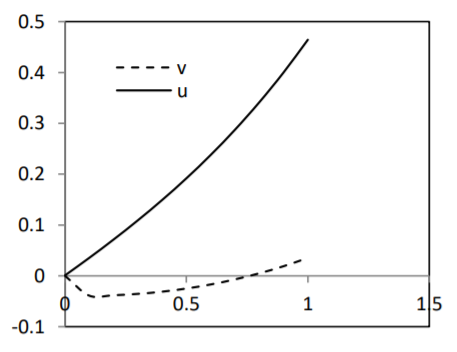Figure 2. M=2, α1=0.5, α2=0.2, ε=0.001, ω=0.5, f=0.1, G=0.8, R=0.2, φ=0.01, mp=0.2, l1=0.5, l2=0.5, Pr=7, Ec=0.01, Lo=0.05

Table 1 is showing the nature of fluid motion for different cases of $\alpha_{1}$ and $\alpha_{2}$ . For smaller values of these two rheological parameters, the energies used in visco-elastic responses are of smaller order of magnitude and hence maximum energy can be reserved and as a response the fluid flows experience acceleration in the motion, i.e, increasing values of $\alpha_{1}$ and $\alpha_{2}$  decelerates the fluid motion. Effect of volume fraction on fluid motion and dust particles are shown in figure 3 and the figures enable the fact that the presence of volume fraction accelerates the fluid motion. Its effect is more prominent on the motion of dust particles, as the magnitude of the speed of dust particles increases but the dust particles experience a back flow in the neighborhood of the lower plate. Figure 4 states that the motion of dust particles reaches steady state quickly then in-comparison to the fluid particles as the change in time creates a negligible variation in the motion of dust particles.

Table 1. M=2, ε=0.001, ω=0.5, f=0.1, G=0.8, R=0.2, φ=0.01, mp=0.2, l1=0.5, l2=0.5, Pr=7, Ec=0.01, Lo=0.05

 Cases u (α1=0.2, α2=0.2) u (α1=0.2, α2=0.5) u (α1=0.5, α2=0.2) y=0 0.0010 0.001 0.001 y=0.1 0.0355 0.035 0.0348 y=0.2 0.0715 0.0704 0.0702 y=0.3 0.1093 0.1076 0.1074 y=0.4 0.1493 0.1472 0.1468 y=0.5 0.1920 0.1895 0.1891 y=0.6 0.2379 0.235 0.2345 y=0.7 0.2875 0.2842 0.2838 y=0.8 0.3414 0.3377 0.3373 y=0.9 0.4001 0.3962 0.3957 y=1 0.4644 0.4601 0.4596

## figure_3.png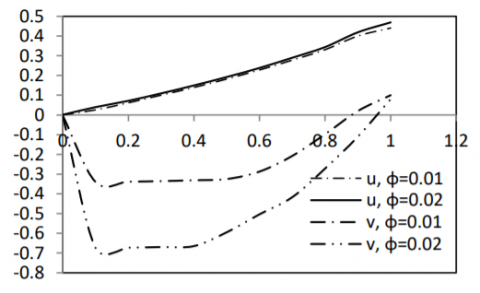Figure 3. M=2, α1=0.5, α2=0.2, ε=0.001, ω=0.5, f=0.1, G=0.8, R=0.2, mp=0.2, l1=0.5, l2=0.5, Pr=7, Ec=0.01, Lo=0.05

## figure_4.png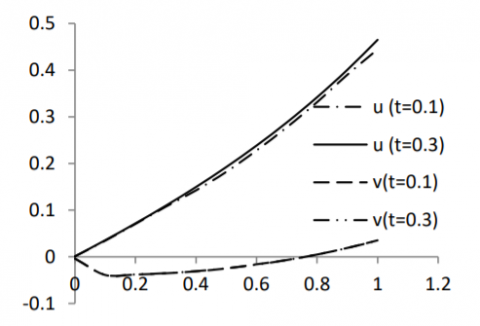Figure 4. M=2, α1=0.5, α2=0.2, ε=0.001, ω=0.5, f=0.1, G=0.8, R=0.2, mp=0.2, φ=0.01, l1=0.5, l2=0.5,  Pr=7, Ec=0.01, Lo=0.05

After studying the velocity profile, now the viscous drag at the surfaces formed by the fluid motion are calculates for various values of flow parameters and it is represented in tabular form. Table 2, states that, magnitude of shearing stresses at both the plates increase with the time but the effect of time is seen prominent at the upper plate.

Effect of relaxation time and retardation time on shearing stress are seen in Table 3a and Table 3b and it is noticed that increase of the relaxation time diminishes the magnitude the of shearing stress at the lower plate.

Table 2. M=2, α1=0.5, α2=0.2, ε=0.001, ω=0.5, f=0.1, G=0.8, R=0.2, mp=0.2, l1=0.5, l2=0.5, Pr=7, Ec=0.01, Lo=0.05

 Cases τ (lower plate) (φ=0.01) τ (upper plate) (φ=0.01) τ (lower plate) (φ=0.02) τ (upper plate) (φ=0.02) t=0.1 2.9325 9.1069 2.9326 9.1074 t=0.2 3.8135 11.5578 3.8136 11.5585 t=0.3 4.6956 13.9910 4.6958 13.9918 t=0.4 5.5766 16.4003 5.5769 16.4012 t=0.5 6.4544 18.7796 6.4547 18.7807 t=0.6 7.3266 21.1232 7.3271 21.1244 t=0.7 8.1912 23.4250 8.1917 23.4264 t=0.8 9.0460 25.6794 9.0465 25.6809 t=0.9 9.8888 27.8807 9.8894 27.8824 t=1 10.7175 30.0234 10.7182 30.0252

Table 3a. M=2, ε=0.001, ω=0.5, f=0.1, G=0.8, R=0.2, φ=0.01, mp=0.2 l1=0.5, l2=0.5, Pr=7, Ec=0.01, Lo=0.05

 Cases (α1=0.2, α2=0.2) (α1=0.2, α2=0.5) (α1=0.5, α2=0.2) τ (lower plate) τ (lower plate) τ (lower plate) 0.1 4.8329 5.6635 2.9325 0.2 5.7999 8.0125 3.8135 0.3 6.7631 10.3520 4.6956 0.4 7.7200 12.6763 5.5766 0.5 8.6683 14.9796 6.4544 0.6 9.6055 17.2561 7.3266 0.7 10.5294 19.5001 8.1912 0.8 11.4376 21.7060 9.0460 0.9 12.3279 23.8683 9.8888 t=1 13.1980 25.9816 10.7175

Table 3b. M=2, ε=0.001, ω=0.5, f=0.1, G=0.8, R=0.2, φ=0.01, mp=0.2, l1=0.5, l2=0.5, Pr=7, Ec=0.01, Lo=0.05

 Cases (α1=0.2, α2=0.2) (α1=0.2, α2=0.5) (α1=0.5, α2=0.2) τ (upper plate) τ (upper plate) τ (upper plate) 0.1 7.5761 5.6635 9.1069 0.2 10.3098 8.0125 11.5578 0.3 13.0288 10.3520 13.9910 0.4 15.7263 12.6763 16.4003 0.5 18.3956 14.9796 18.7796 0.6 21.0300 17.2561 21.1232 0.7 23.6230 19.5001 23.4250 0.8 26.1680 21.7060 25.6794 0.9 28.6587 23.8683 27.8807 1 31.0888 25.9816 30.0234

The same diminishing effect of retardation time is seen on the shearing stress at the upper plate but it has an increasing effect at the lower plate.Volume fraction also has a positive impact on shearing stresses at both the plates but its effect is seen superior at the upper plate (Table 2).

Both the temperatures of fluid particles and dust particles rise in the neighbourhood of the upper oscillating plate (figure 5). Effects of relaxation time and retardation time on the temperature fields are represented by Table 4 and Table 5 and it is experienced that during the growth of relaxation time, the temperature of dusty visco-elastic fluid and dust particles experience enhancing pattern over the entire channel but a reverse mechanism is seen during the growth of retardation parameter.

Nusselt number plays an important role in the mechanism of heat transfer as it enables the rate of heat transfer in the governing fluid motion. Table 6 is representing the numerical values of Nusselt number at the plates for a time period [0.1, 1] and it can be concluded that magnitude of rate of heat transfer increases in the above mentioned time period at both the plates.

## figure_5.png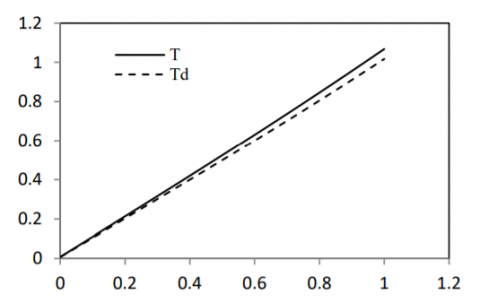Figure 5. M=2, α1=0.5, α2=0.2, ε=0.001, ω=0.5, f=0.1, G=0.8, R=0.2, φ=0.01, mp=0.2, l1=0.5, l2=0.5,  Pr=7, Ec=0.01, Lo=0.05

Table 4. M=2, α1=0.5, α2=0.2, ε=0.001, ω=0.5, f=0.1, G=0.8, R=0.2, φ=0.01, mp=0.2, l1=0.5, l2=0.5, Pr=7, Ec=0.01, Lo=0.05

 Cases T (α1=0.2, α2=0.2) T (α1=0.2, α2=0.5) T (α1=0.5, α2=0.2) y=0 0.0078 0.0077 0.008 y=0.1 0.1102 0.1101 0.1104 y=0.2 0.2128 0.2127 0.2131 y=0.3 0.3159 0.3158 0.3161 y=0.4 0.4196 0.4195 0.4199 y=0.5 0.5242 0.5241 0.5245 y=0.6 0.6299 0.6298 0.6302 y=0.7 0.7369 0.7368 0.7372 y=0.8 0.8454 0.8453 0.8457 y=0.9 0.9557 0.9556 0.956 y=1 1.0679 1.0678 1.0682

Table 5. M=2, ε=0.001, ω=0.5, f=0.1, G=0.8, R=0.2, φ=0.01, mp=0.2,  l1=0.5, l2=0.5, Pr=7, Ec=0.01, Lo=0.05

 Cases Tp(α1=0.2,α2=0.2) Tp(α1=0.2,α2=0.5) Tp(α1=0.5,α2=0.2) y=0 0.0074 0.0073 0.0076 y=0.1 0.1049 0.1048 0.1052 y=0.2 0.2027 0.2026 0.2029 y=0.3 0.3008 0.3007 0.3011 y=0.4 0.3996 0.3995 0.3999 y=0.5 0.4992 0.4991 0.4995 y=0.6 0.5999 0.5998 0.6002 y=0.7 0.7018 0.7017 0.7021 y=0.8 0.8052 0.8051 0.8054 y=0.9 0.9102 0.9101 0.9105 y=1 1.0171 1.0170 1.0174

Table 6. M=2, α1=0.5, α2=0.2, ε=0.001, ω=0.5, f=0.1, G=0.8, R=0.2, φ=0.01, mp=0.2,  l1=0.5, l2=0.5, Pr=7, Ec=0.01, Lo=0.05

 Cases Nu (lower plate) Nu (upper plate) t=0.1 0.9504 0.9349 t=0.2 0.9507 0.9353 t=0.3 0.9511 0.9369 t=0.4 0.9518 0.9367 t=0.5 0.9526 0.9378 t=0.6 0.9536 0.09391 t=0.7 0.9548 0.9407 t=0.8 0.9562 0.9424 t=0.9 0.9577 0.9444 t=1 0.9594 0.9467
5. Conclusions

Some of the important conclusions from the above work are cited below:

• Speed of fluid flow is maximum in the neighbourhood of the upper plate.
• Dust particles experience a back flow in the neighbourhood of the lower plate.
• Increasing values of relaxation and retardation time parameters decelerates the fluid motion.
• Temperature of fluid and dust particles increases uniformly from lower surface to upper surface.
• Magnitude of Nusselt number increases in the time interval [0.1, 1] at both the plates.
Acknowledgment

The author acknowledges Professor Rita Choudhury, Department of Mathematics, Gauhati University for her constant encouragement throughout the work

Nomenclature
 vi, $u^{\prime}$ Velcoity of fluid, LT-1 vpi, $v^{\prime}$ Velocity of dust in tensorial form, LT-1 xi, $x^{\prime}$ $t^{\prime}$ Displacement variable, L Time, T p d Ji K= 6πµa $T^{\prime}$ $T^{\prime} p$ Cp Cp N mp k Bi U0 y u v t T Tp f R M G Pr Ec Nu Sh L0 Fluid pressure, ML-1T-2 Distance between two plates, L Current density, IL-2 Stokes constant, MT-1 Temperature of fluid, K(Kelvin) Temperature of dust particles, K Specific heat of fluid at constant pressure, L2T-2K-1 Specific heat of dust at constant pressure, L2T-2K-1 Number of dust particles per unit volume, L-3 Average mass of dust particles, M Thermal conducitivity, MLT-3I2 Magnetic induction vector, MT-2I-1 A constant, LT-1 Dimensionless displacement variable Dimensionless velocity of fluid Dimensionless velocity of dust particles Dimensionless time Dimensionless temperature of fluid Dimensionless temperature of dust Particle concentration parameter Reynolds number  Hartmann number Particle mass parameter Prandtl number Eckert number Nusselt number Shearing stress Dimensionless temperature relaxation time Greek symbols $\rho$ $\rho_{0}$ $\tau_{i j}, \tau$ $\mathcal{V}$ $\eta_{0}$ $\sigma$ $\mathcal{E}$ $\lambda_{1}$ $\lambda_{2}$ $\alpha_{1}$ $\alpha_{2}$ $\phi$ $\gamma_{T}$ $\omega^{\prime}$ $\omega$ $\varepsilon_{\mathrm{ijk}}$ Density of fluid, ML-3 Density of dust particle, ML-3 Viscous stress, ML-1T-2 Kinematic viscosity, L2T-1 Dynamic viscosity, ML-1T-1 Electrical conductivity, L-3M-1T3I2 Dimensionless amplitude of oscillation Relaxation time parameter, T Retardation time parameter, T Dimensionless relaxation time Dimensionless retardation time Volume fraction Temperature relaxation time, T Frequency of oscillation, T-1 Dimensionless frequency Levi-Civita symbol
References

 Oldroyd, J. G., “On the Formulation of Rheological Equations of State,” Proceedings of the Royal Society of London. Series A, Mathematical and Physical Sciences, vol. 200, no. 1063, pp. 523–541, 1950. DOI: 10.1098/rspa.1950.0035.

 Oldroyd, J. G., “Non-Newtonian effects in steady motion of some idealized elastic-viscous liquids,” Proc. Roy. Soc. Lond. A, vol. 245, no. 1241, pp. 278-297, 1958. DOI: 10.1098/rspa.1958.0083.

 Sellers, C. C. and Walker, J. S., “Liquid-metal in an electrically insulated rectangular duct with a non-uniform magnetic field,” Int. J. Engg. Sci.,vol. 37, pp. 541-552, 1999. DOI: 10.1016/S0020-7225(98)00088-3.

 Mukhopadhyay, D. N. and Chaudhury, T. K., “On the flow of visco-elastic liquids past an infinite porous plate due to fluctuation in the main flow,” ASME, J. Appl. Mech., vol. 49, no. 3, pp. 644-646, 1982. DOI: 10.1115/1.3162543.

 Rajagopal, K. R. and Bhatnagar, R. K., “Exact solutions for some simple flows of an Oldroyd-B fluid,” Acta Mechanica, vol. 113, pp. 233-239, 1995. DOI: 10.1007/BF01212645.

 Ray, R. N.,  Samad, A. and Chaudhury, T. K., “An exact periodic solution of hydro-magnetic flow of an Oldroyd fluid in a channel,” ASME, J. Appl. Mech., vol. 66, no. 4, pp. 974-977, 1999. DOI: 10.1115/1.2791807.

 Hayat, T., Siddiqui, A. M., and Asghar, S., “Some simple flows of an Oldroyd-B fuid,” International Journal of Engineering Science, vol. 39, pp. 135-147, 2001. DOI: 10.1016/S0020-7225(00)00026-4.

 Hayat, T., Nadeem, S. and Asghar, S., “Hydromagnetic couette flow of an Oldroyd-B fluid in a rotating system,” International Journal of Engineering Science, vol. 42, pp. 65–78, 2004. DOI: 10.1016/S0020-7225(03)00277-5.

 Asghar, S., Parveen, S., Hanif, S. and Siddique, A. M., “Hall effects on the unsteady hydromagnetic flows of an Oldroyd-B fluid,” Int. J. Engg. Sci., vol. 41, pp. 609-619, 2004. DOI: 10.1016/S0020-7225(02)00153-2.

 Hayat, T., Wang, Y., and Hutter, K., “Hall effects on the unsteady hydromagnetic oscillatory flow of a second grade fluid,” Int. J. Non Linear Mech., vol. 39, pp. 1027-1037, 2004. DOI: 10.1016/S0020-7462(03)00094-5.

 Prasad, V., Nagaich, M., and Varshney, N. K.,  “Effect of oldroyd fluid on unsteady laminar free convective flow along a moving porous hot vertical plate with thermal diffusion and mass transfer,” International Journal of Mathematical Archive, vol. 3, no. 11, pp.  4718-4724, 2012.

 Ghosh, S. K., “Unsteady hydro-magnetic flow of an Oldroyd fluid through a porous channel with oscillating walls,” Journal of Physical Sciences, vol. 17, pp. 155-167, 2013.

 Choudhury, R. and Das, U. J., “Visco-elastic effects on the three dimensional hydromagnetic flow past a vertical porous plate,” Int. J. Heat Tech., vol. 31, no. 1, pp. 1-8, 2013.

 Choudhury, R. and Purkayastha, S., “An analysis of mixed convective elastic-viscous fluid past a vertical porous plate in presence of induced magnetic field and chemical reaction,” Int. J. Heat Tech., vol. 32, no. 1-2, pp. 27-34, 2014.

 Choudhury, R. and Das, B., “Influence of visco-elasticity on MHD heat and mass transfer flow through a porous medium bounded by an inclined surface with chemical reaction,” Int. J. Heat Tech., vol. 34, no. 2, pp. 332-338, 2016.

 Saffman P. G., “On the stability of laminar flow of a dusty gas,” J. Fluid Mech., vol. 13, pp. 120-128, 1962. DOI: 10.1017/S0022112062000555.

 Michael, D. H. and Miller, D. A., “Plane parallel flow of a dusty gas,” Mathematika, vol. 13, pp. 97-109, 1966. DOI:  10.1112/S0025579300004289.

 Rudinger, G., “Some effects of finite particle volume on the dynamics of gas-particle mixtures,” AIAA Journal, vol. 3, pp. 1217-1222, 1965. DOI: 10.2514/3.3112.

 Nayfeh A. H., “Oscillating two-phase flow through a rigid pipe,” AIAAJ, vol. 4 no. 10, pp. 1868-1870, 1966. DOI: 10.2514/3.3804.

 Gupta, R. K. and Gupta, S. C., “Flow of a dusty gas through a channel with arbitrary time varying pressure gradient,” Journal of Appl. Math. and Phys., vol. 27, pp. 119-125, 1976. DOI: 10.1007/BF01595248.

 Singh K. K., “Unsteady flow of a conducting dusty fluid through a rectangular channel with time dependent pressure gradient,” Indian J. Pure and Appl. Math., vol. 8, pp. 1124-1131, 1976.

 Singh, C. B. and Ram, P. C., “Unsteady flow of an electrically conducting dusty viscous liquid through a channel,” Indian J. Pure and Appl. Math., vol. 8, no. 9, pp. 1022-1028, 1977.

 Prasad, V. R. and Ramacharyulu, N. C. P., “Unsteady flow of a dusty incompressible fluid between two parallel plates under an impulsive pressure gradient,” Def. Sci. Journal, vol. 38, pp. 125-130, 1979.

 Gupta, R. K. and Gupta K., “Unsteady flow of a dusty non-Newtonian fluid through channel with volume fraction,” Indian J. Pure and Appl. Math., vol. 21, no. 7, pp. 677-690, 1990.

 Ajadi, S. O., “A note on the unsteady flow of dusty viscous fluid between two parallel plates,” J. Appl. Math. and Computing, vol. 18, no. 1, pp. 393-403, 2005. DOI: 10.1007/BF02936582.

 Attia, H. A.,  Al-Kaisy, A. M. A. and Ewis, K. M.,  “MHD Couette flow and heat transfer of a dusty fluid with exponential decaying pressure gradient,” Tamkang J. Sci. and Engg., vol. 14, no. 2, pp. 91-96, 2011.

 Kumar, N. and Gupta, S., “MHD free convection and entrpy generation in a circular channel occupied by hyper poroud medium,” Int. J. Heat Tech., vol. 29, no. 1, pp. 91-100, 2011.

 Kumar, N., Gupta, S. and Jain, T. “Effects of thermal radiation and mass transfer on MHD unsteady flow through a porous medium bounded by two porous horizontal parallel plates moving in opposite directions,” Int. J. Heat Tech., vol. 29, no. 2, pp. 41-50, 2011.

 Ali, N., Khan, S. U., Sajid, M. and Abbas, Z., “Flow and heat transfer of hydromagnetic Oldroyd-B fluid in a channel with stretching walls,” Non-linear Engineering, vol. 5, no. 2, pp. 73-39, 2016. DOI: 10.1515/nleng-2015-0035.

 Hemorheology. N. D. In Wikipedia. Retrived August 23, 2016, from https://en.wikipedia.org/wiki/ Hemorheology#Blood_viscoelasticity